Next: Spin Precession Up: Spin Angular Momentum Previous: Rotation Operators in Spin

# Magnetic Moments

Consider a particle of electric charge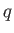and speed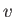performing a circular orbit of radiusin the-plane. The charge is equivalent to a current loop of radius, lying in the-plane, and carrying a current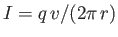. The magnetic moment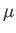of the loop is of magnitude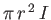and is directed along the-axis (the direction is given by a right-hand rule with the fingers of the right-hand circulating in the same direction as the current) . Thus, we can write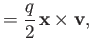(5.42)

where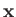and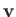are the vector position and velocity of the particle, respectively. However, we know that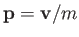, where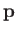is the particle's vector momentum, and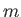its mass. We also know that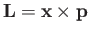, where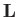is the orbital angular momentum. It follows that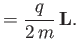(5.43)

Using the standard analogy between classical and quantum mechanics, we expect the previous relation to also hold between the quantum mechanical operators,and, which represent magnetic moment and orbital angular momentum, respectively. This is indeed found to the the case experimentally.

Spin angular momentum also gives rise to a contribution to the magnetic moment of a charged particle. In fact, relativistic quantum mechanics predicts that a charged particle possessing spin must also possess a corresponding magnetic moment [30,32,9]. We can write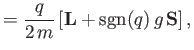(5.44)

where the parameter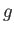is called the-factor. For an electron this factor is found to be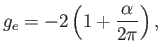(5.45)

where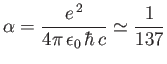(5.46)

is the fine structure constant. The factor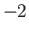is correctly predicted by Dirac's famous relativistic theory of the electron [30,32]. (See Chapter 11.) The small correction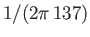is due to quantum field effects . We shall ignore this correction in the following, so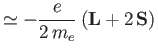(5.47)

for an electron. (Here,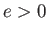is the magnitude of the electron charge, and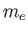the electron mass.)Next: Spin Precession Up: Spin Angular Momentum Previous: Rotation Operators in Spin
Richard Fitzpatrick 2016-01-22